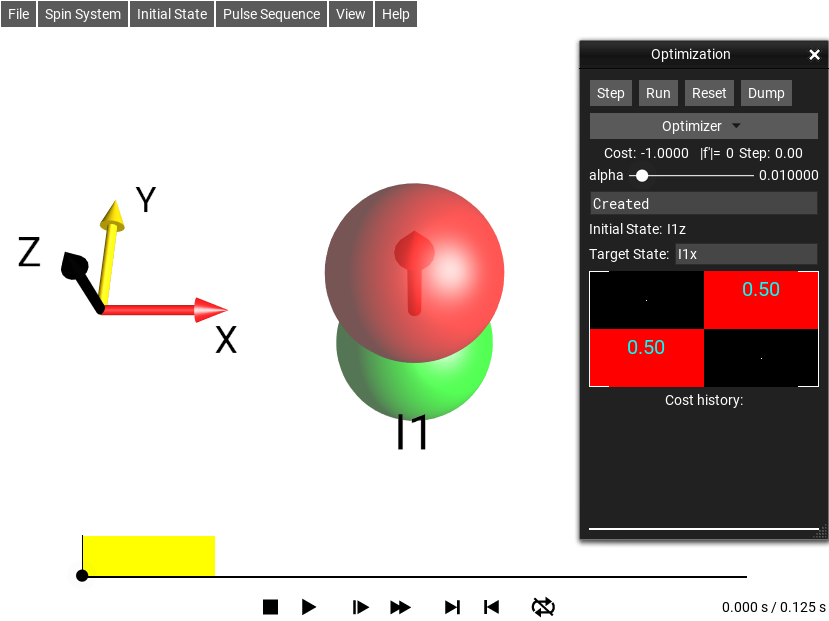# Sequence Optimizer¶

The menu selection Pulse Sequence ‣ Sequence Optimizer… opens the Optimization window. This window presents optimization tools that can modify the currently loaded sequence to perform a particular state transformation from the selected Initial State to a Final State, which can be defined by the user in this window. The current implementation is very simplistic, and simply performs a fixex-step gradient descent type of optimization.Fig. 183 The sequence Optimization window.

## Buttons¶

The pulse optimization tool runs in real-time so you can watch the progress of iterative optimization while it is being calculated. Because the algorithms are (so far) iterative, the current state is displayed each iteration. The Step button performs a single iteration, while the Run button lets the optimization iterate repeatedly until it has achieved its convergence (or its “give up”) criteria. If the optimization is running, clicking Run again stops the running optimization.

The Reset button resets the sequence or pulse to the un-optimized initial sequence parameters. The optimization algorithm is designed to only optimize elements of the pulse sequence which it determines to be ‘pulses’, and to ignore delay periods.

Finally, the optimization result can be exported from SpinDrops by using the Dump button. The exported file contains a number of matrices in a format which can be copy/pasted into Octave or Matlab.

The dump file optim.txt file contains the following matrices:

• x

This is the calculated optimal pulse sequence matrix. Each column is a time-step, and each row is a different control parameter. The first rows are pairs of {x,y} RF channels corresponding to the RF operators in the experiment. After the RF channels come any user-defined Hamiltonian channels, and finally the last row is always the time-duration (in seconds) of the column.

• dx

This is the partial derivatives of the cost fuction with respect to the controls x. When the optimized value of x is truly optimal, these should be very very close to zero. In case of reaching the undesired local minimum (as opposed to global minimum), these values could also be very close to zero.

• xmin

This matrix contains the constraints on the lower values of x.

• xmax

This matrix contains the constraints on the upper values of x.

• xon

This is a boolean matrix indicating which values of x should be considered in the optimization. This is currently calculated by determining which controls in x are pulse elements.

• acqN

If the pulse sequence has an acquisition element (either a table-sequence Table Acq, or go, each of the acquisitions (not traces) will also be included in the dump.